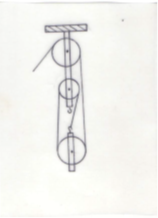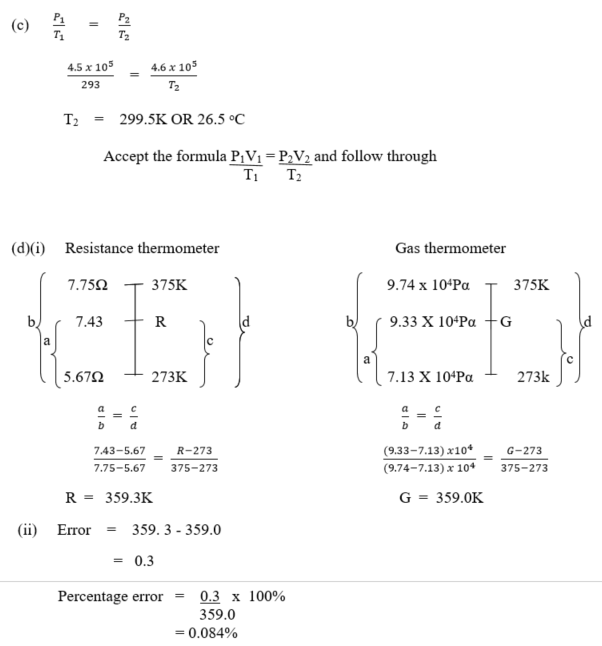Question 9

1. Define stable equilibrium as applied to a rigid body
2. Sketch a block and tackle system of pulleys with a velocity ratio of 3
3. At the beginning of a race, a tyre of volume 8.0 x 10-4 m3 at 20oC has a gas pressure of 4.5 x 105 Pa. Calculate the temperature of the gas in the tyre at the end of the race if the pressure has risen to 4.6 x 105 Pa.
4. (i)
 Ice Point 273K Steam Point 373K Resistance/Ω 5.67 7.75 Pressure/Pa 7.13 x 104 9.74 x 104

The table above shows readings of the resistance and pressure of a platinum resistance thermometer and a constant volume gas thermometer respectively when immersed in the same liquid bath. Use these data to determine the temperature of the bath on the
(α) resistance thermometer;
(β) gas thermometer;
When the resistance and pressure are 7.43 Ω and 9.33 x 104 Pa respectively.

(ii) By what percentage is the temperature measured on the platinum resistance thermometer in error?

Observation

Part (a): Quite a good number candidates got acceptable response to score full marks. Some
candidates ignored the need for the displacement to be “slight”. That resulted
into zero instead of the full mark. Performance was fair.
Part (b): This question was a popular question but majority of the candidates scored zero because of incorrect rope looping. A correct sketch must show three pulleys, two fixed and one moveable and a rope correctly fixed. Performance was low
Part (c):  The performance of candidates was satisfactory. Although some candidates failed
to change the initial temperature to value in Kelvin for the Physics to be correct.
Part(d):   Majority of candidates who attempted this questions skipped due to
incompleteness of the question.

9.  (a)  Stable equilibrium

A body is said to be in stable equilibrium if it tends to return to its original position after being slightly displaced.

(B)Correct sketch showing:

• Three pulleys; two fixed and one movable
• Rope correctly fixed